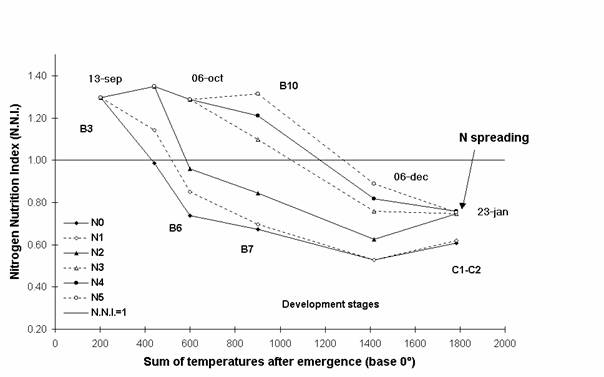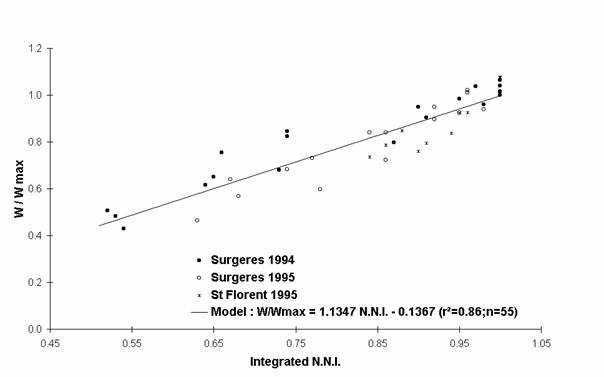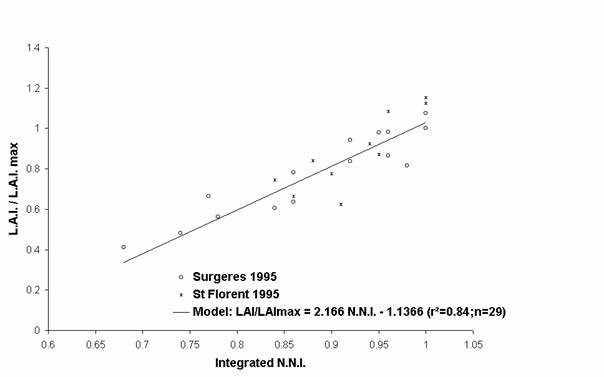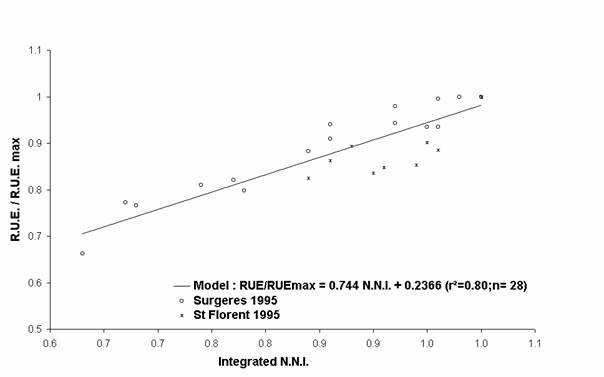Effects of different temporary nitrogen deficiencies in Autumn on winter oilseed rape growth : aerial biomass , leaf area index and radiation use efficiency.

### C. Colnenne 1 and JM. Meynard 2

1 Institut Supérieur d’Agriculture de Lille, F59046 Lille cedex Colnenne@isa.fupl.asso.fr

2 Institut National de la Recherche Agronomique, F78850 Thiverval-Grignon, Meynard@jouy.inra.fr

#### ABSTRACT

In the model CERES CECOL, winter oilseed rape growth is simulated according to the concept developed by Monteith (1972). Different field experiments have been carried out to analyse the effects of various nitrogen deficiencies in Autumn on the different growth components. Precise models have been defined between the nitrogen status of the crop and the evolution of (1) the shoot biomass, (2) the L.A.I. and (3) the R.U.E.. They could be utilised in CERES CECOL take into account the nitrogen status of the crop in Autumn.

Keywords : Nitrogen Nutrition Index, model, CERES CECOL

Introduction

The winter oilseed rape growth  model CERES CECOL is made up of two modules. One takes into account the evolution of the soil compartment, the other the growth and development of the crop (Husson, 1997). In this second part, the growth increase is simulated according the model developed by Monteith (1972) where W = PAR * Ei * R.U.E.. This concept describes the growth (W) as a function of (1) the Radiation Interception Efficiency (Ei), depending on L.A.I., (2) the Radiation Use Efficiency (R.U.E.). This approach allows us to prioritize the consequences of various limiting growth conditions (temperature, water or mineral nutrition stress ect…) or effects of agricultural practices (sowing date, density ect…) which can occur at different levels of the dry matter elaboration process.

In Autumn and in Winter the winter oilseed rape presents, in N limiting nutrition conditions, an important loss and some modifications of these leaves. In consequence, an analytical study has been carried out to specifically determine the consequences of Autumn N deficiencies, characterized by the integrated N.N.I. (Lemaire et al., 1997) on each of those different growth components (i.e. L.A.I. and R.U.E.).

1. Materials and methods

Three field experiments with the winter oilseed rape cultivar Goeland were carried out during two winter growing seasons (1994, 1995) in different pedoclimatic conditions. To obtain different Autumn nitrogen deficiencies, the sowings were early in Autumn, following an underfertilizered crop and on subsurface soil. Each experiment included six Autumn nitrogen fertilizer levels (0, 20, 40, 60, 80 and 100 kg N /ha amonium nitrate) at emergence. In Spring, the plants were fertilized perhaps even superfertilized to prevent a lack of nitrogen during this period.

In Autumn we took regular measurements of dry matter, total nitrogen concentration (Dumas Chemical analysis) on the aerial parts and the L.A.I. on three samples of 0.8 m² from each plot. The data collected in Autumn allowed us to calculate the Nitrogen Nutrition Index (Lemaire et al., 1989) and meteorology data have been collected to calculate the Radiation Interception Efficiency (Ei).

2. Results

2.1. Evolution of the Nitrogen deficiencies.

We chose to present the results from one experiment Surgeres in 1995 (cf. Figure 1). We can see, in Figure 1, the values of the N.N.I. between 13 September 1994 (3 green leaves stage) and 23 January 1995. The nitrogen deficiency of the 0 kg N /ha treatment became approximately 450° (base 0) earlier than the 100 kg N/ha one, and was stronger. At the last observation, the N.N.I. values were 0.56 (which is very low) and 0.83 for the 0 kg N /ha and 100 kg N /ha respectively.

Figure 1 : Evolution of the nitrogen nutrition index : Surgeres 1995To take into account both the duration and the intensity of the nitrogen deficiencies in Autumn, we chose to characterize the nitrogen stress with the integrated N.N.I. (Lemaire et al., 1997). This index corresponds to the ratio beetwen the integrated N.N.I. values calculated on one treatment during a defined period and the duration of this period.

2.2. Consequences of the nitrogen deficiencies on growth components.

To cancel out from the different pedoclimatic conditions specific to each experiment, the effects of nitrogen deficiencies have been analysed on growth components expressed in relative values. For example, the variable W/Wmax corresponds to the ratio between the shoot biomass of each treatment (W) and the maximum shoot biomass value measured at the same time (Wmax). Wmax is the mean of the maximum measurements defined by the statistic test Newman and Keuls, at 5%. The L.A.I./ L.A.I.max and the R.U.E./R.U.E.max ratio have been calculated  with the same methodology.

The evolution of each relative growth variable has been represented according to the nitrogen status of plants in Figures 2, 3 and 4. Each growth variable is well correlated with the nitrogen status of the crop. Earlier and stronger the nitrogen deficiencies were, higher the decrease of each relative growth variable was. All these relative growth data have been well fitted by simple linear regressions (model equations, and determination coefficients appear in Figures 2, 3 and 4). For an integrated N.N.I. equal to 0.70, the shoot biomass reduction of 34% can be explained by both the decrease of the L.A.I. (- 62%) and the R.U.E. (- 24%)

Conclusion

The Autumn nitrogen deficiencies have pronounced consequences either on aerial growth on the L.A.I. (i.e. on Ei) and on the R.U.E.. For winter oilseed rape, in such nitrogen limiting growth conditions, the decrease of the shoot dry matter is explained by a higher reduction of the L.A.I. than the reduction of the R.U.E..

This analysis allows us to compare the responses of different species in nitrogen limiting growth conditions and understand their specific behaviors (for example fescue : Belanger et al. (1992), or maize : Plenet et Cruz, 1997).

On the other hand, these findings can be used in different models, such as CERES CECOL or AZODYN, and more accurately take into account the consequences of nitrogen deficiencies on the growth.

Figure 2 : Evolution of the shoot weight with nitrogen deficienciesFigure 3 : Evolution of the relative L.A.I. with the nitrogen deficienciesFigure 4 : Evolution of the relative R.U.E. with the nitrogen deficienciesAcknowledgements

We thank the members of CETIOM for theirs participations in data collecting, advice, and the CETIOM which finances the experiments.

###### References

Belanger G., Gastal F., Lemaire G., 1992. Growth analysis of a tall fescue sward fertilized with different rates of nitrogen. Crop Sciences , 32 (1371-1376).

Husson F., Leterme P., 1997. Construction et validation d’un modèle de prédiction de la date de floraison du colza d’hiver. O.C.L., 5 (379-384).

Lemaire G., Meynard J.M., 1997. Use of the nitrogen nutrition index for the analysis of agronomical data. In : Lemaire G. ed. Diagnosis of the nitrogen status in crops. Berlin Heidelberg : Springer-Verlag, (45-55).

Monteith J.L., 1972. Solar radiation and productivity in tropical ecosystems. Journal of Applied Ecology, 9 (747-766).

Plenet D., Cruz P., 1997. The nitrogen requirement of major agricultural crops : Maize and sorghum.. In : Lemaire G. ed. Diagnosis of the nitrogen status in crops. Berlin Heidelberg : Springer-Verlag, (93-107).